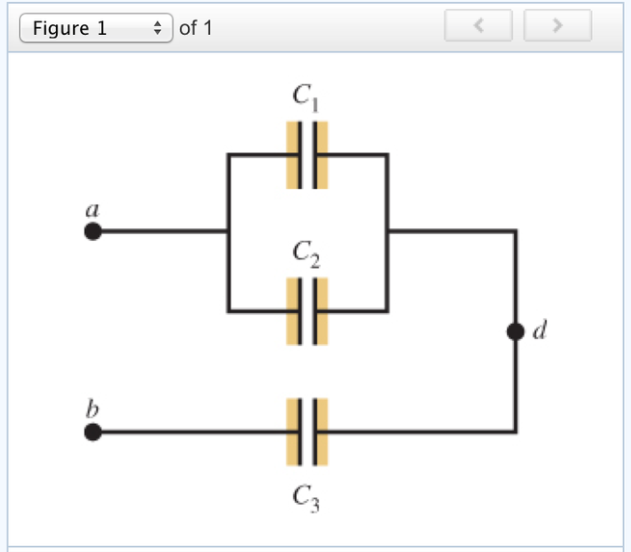# Problem: In the figure are shown three capacitors with capacitances C1 = 6.04 × 10-6 F, C2 = 3.6 × 10-6 F, and C3 = 4.47 × 10-6 F. The capacitor network is connected to an applied potential Vab. After the charges on the capacitors have reached their final values, the charge on the second capacitor is 4.23 × 10-5 C.(a) What is the charge Q1 on capacitor C1? (b) What is the charge on capacitor C3? (c) What is the applied voltage, Vab?

###### FREE Expert Solution

The charge stored on a capacitor:

$\overline{){\mathbf{Q}}{\mathbf{=}}{\mathbf{C}}{\mathbf{V}}}$

(a)

Q2 = 4.23 × 10-5 C

C2 = 3.6 × 10-6F

V2 = Q2/C2 = (4.23 × 10-5)/(3.6 × 10-6) = 11.75 V

Voltage across parallel capacitors is the same. C1 and C2 are in parallel.

100% (147 ratings)###### Problem Details

In the figure are shown three capacitors with capacitances C1 = 6.04 × 10-6 F, C2 = 3.6 × 10-6 F, and C3 = 4.47 × 10-6 F. The capacitor network is connected to an applied potential Vab. After the charges on the capacitors have reached their final values, the charge on the second capacitor is 4.23 × 10-5 C.(a) What is the charge Q1 on capacitor C1

(b) What is the charge on capacitor C3

(c) What is the applied voltage, Vab?